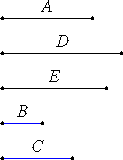# Proposition 10

To find two straight lines incommensurable, the one in length only, and the other in square also, with an assigned straight line.

Let A be the assigned straight line.

It is required to find two straight lines incommensurable, the one in length only, and the other in square also, with A.

X.6,Cor.

Set out two numbers B and C which do not have to one another the ratio which a square number has to a square number, that is, which are not similar plane numbers, and let it be contrived that B is to C as the square on A is to the square on D, for we have learned how to do this.X.6

Therefore the square on A is commensurable with the square on D.

X.9

And, since B does not have to C the ratio which a square number has to a square number, therefore neither has the square on A to the square on D the ratio which a square number has to a square number, therefore A is incommensurable in length with D.

V.Def.9

Take a mean proportional E between A and D. Then A is to D as the square on A is to the square on E.

X.11

But A is incommensurable in length with D, therefore the square on A is also incommensurable with the square on E. Therefore A is incommensurable in square with E.

Therefore two straight lines D and E have been found incommensurable, D in length only, and E in square and of course in length also, with the assigned straight line A.

Q.E.D.

## Guide

This proposition exhibits the lines promised in X.Def.I.3. Just take a line D so that the square on A to the square on D is the ratio of two numbers which are not a square number to a square number. For instance, if A is the side of a square and D the diagonal of that square, then the square on A to the square on D is in the ratio 1:2, which is not the ratio of square number to a square number. Therefore D is commensurable in square only with A. (The ratio D : A is the square root of 2.)

Next, if E is the mean proportional between A and D, then E is incommensurable in square with A. (The ratio E : A is the fourth root of 2.)

It is certain that this proposition is not genuine. For one thing, its proof uses the next proposition. Also, the phrase “for we have learned how to do this” is the sort of thing a student would write. Finally, in the manuscript P (the primary one used by Peyrard and Heiberg) this proposition is not numbered and the next one is numbered 10.

Although not genuine, this proposition ought to be, since it is used in proposition X.27 and others.